Скачать презентацию CS 259 Winter 2008 Cryptography Overview John Mitchell

2f857dbb662c5149b2eb696af1308125.ppt

• Количество слайдов: 45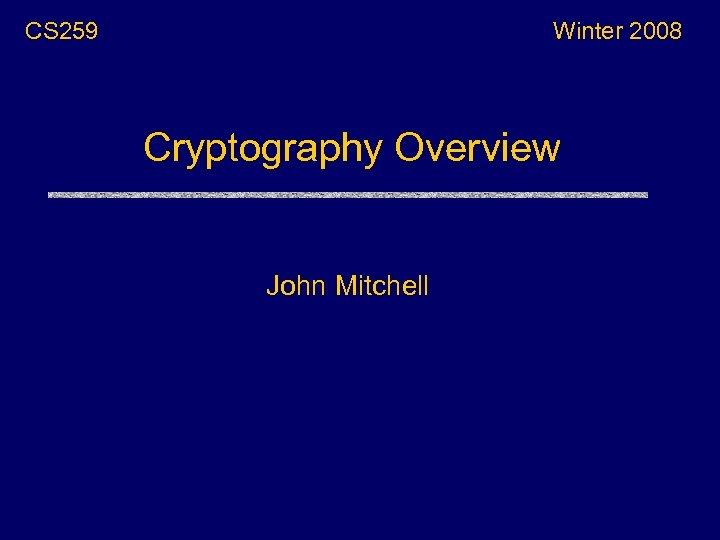CS 259 Winter 2008 Cryptography Overview John Mitchell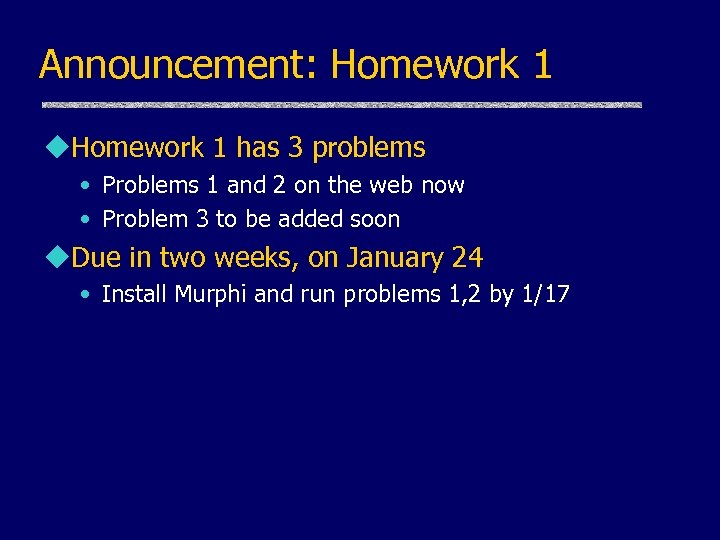Announcement: Homework 1 u. Homework 1 has 3 problems • Problems 1 and 2 on the web now • Problem 3 to be added soon u. Due in two weeks, on January 24 • Install Murphi and run problems 1, 2 by 1/17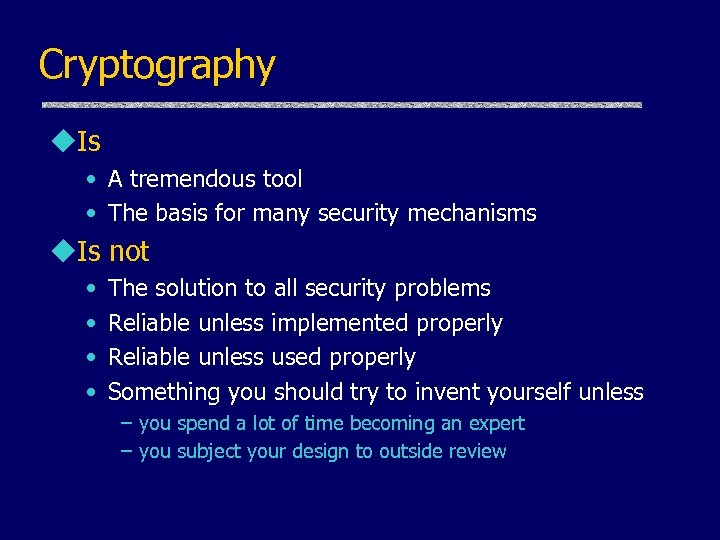Cryptography u. Is • A tremendous tool • The basis for many security mechanisms u. Is not • • The solution to all security problems Reliable unless implemented properly Reliable unless used properly Something you should try to invent yourself unless – you spend a lot of time becoming an expert – you subject your design to outside review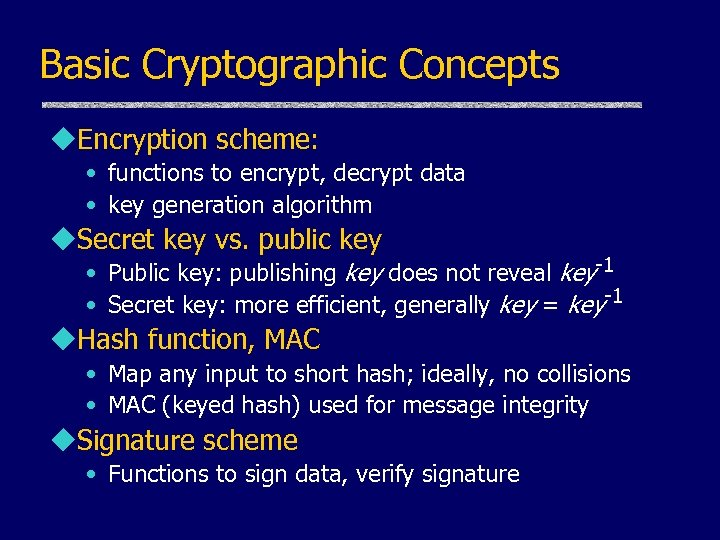Basic Cryptographic Concepts u. Encryption scheme: • functions to encrypt, decrypt data • key generation algorithm u. Secret key vs. public key • Public key: publishing key does not reveal key-1 • Secret key: more efficient, generally key = key-1 u. Hash function, MAC • Map any input to short hash; ideally, no collisions • MAC (keyed hash) used for message integrity u. Signature scheme • Functions to sign data, verify signature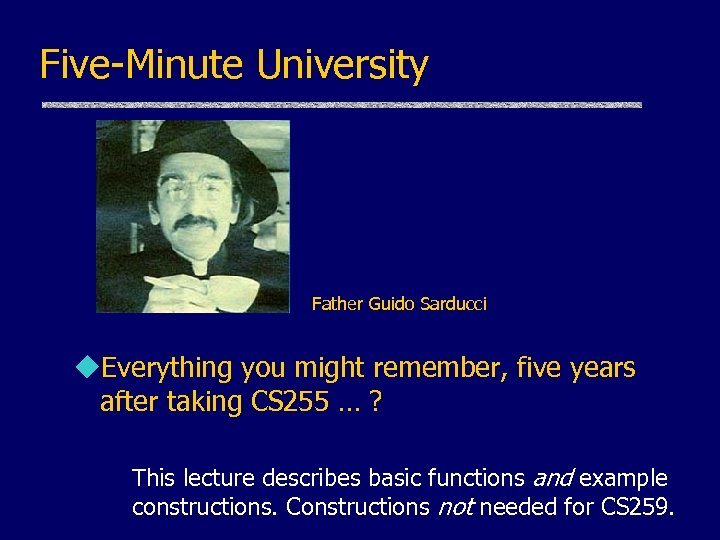Five-Minute University Father Guido Sarducci u. Everything you might remember, five years after taking CS 255 … ? This lecture describes basic functions and example constructions. Constructions not needed for CS 259.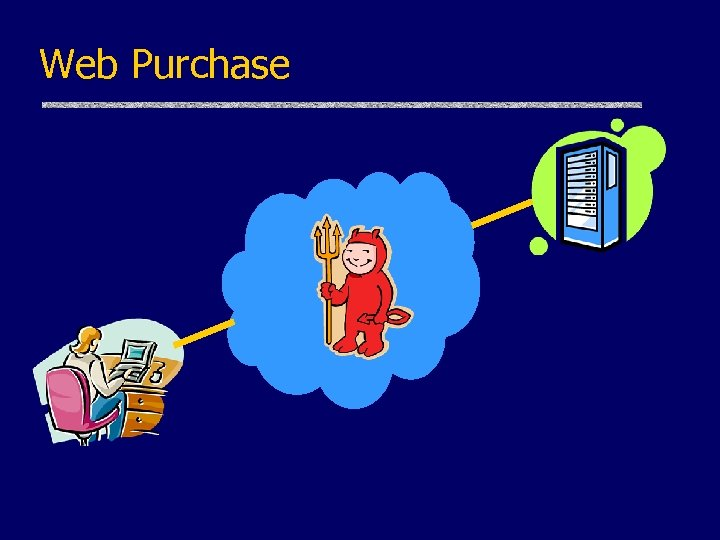Web Purchase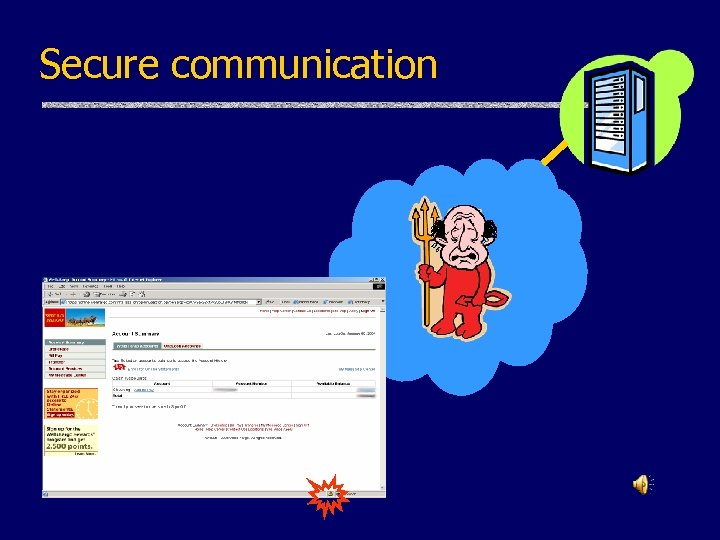Secure communication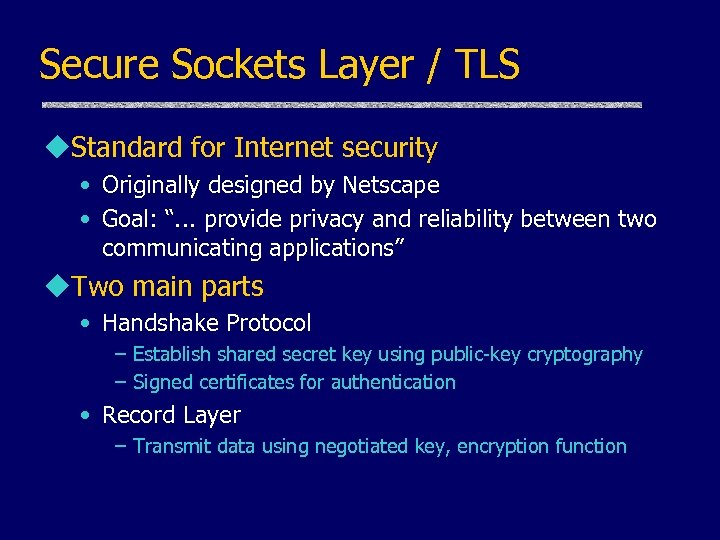Secure Sockets Layer / TLS u. Standard for Internet security • Originally designed by Netscape • Goal: “. . . provide privacy and reliability between two communicating applications” u. Two main parts • Handshake Protocol – Establish shared secret key using public-key cryptography – Signed certificates for authentication • Record Layer – Transmit data using negotiated key, encryption function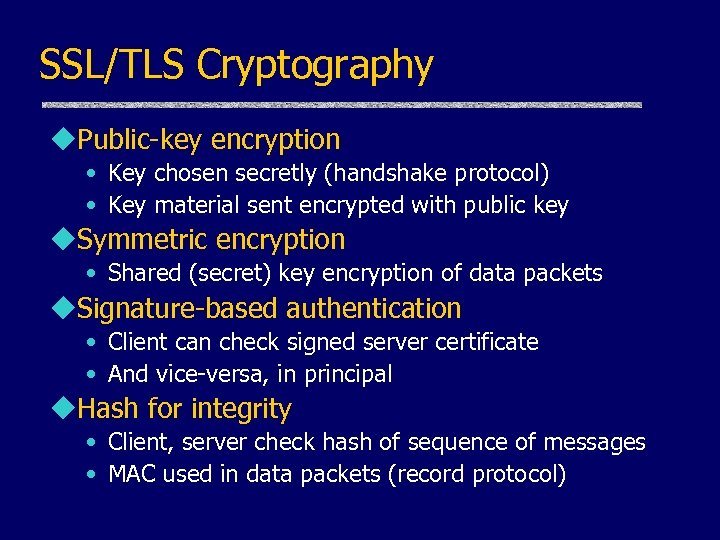SSL/TLS Cryptography u. Public-key encryption • Key chosen secretly (handshake protocol) • Key material sent encrypted with public key u. Symmetric encryption • Shared (secret) key encryption of data packets u. Signature-based authentication • Client can check signed server certificate • And vice-versa, in principal u. Hash for integrity • Client, server check hash of sequence of messages • MAC used in data packets (record protocol)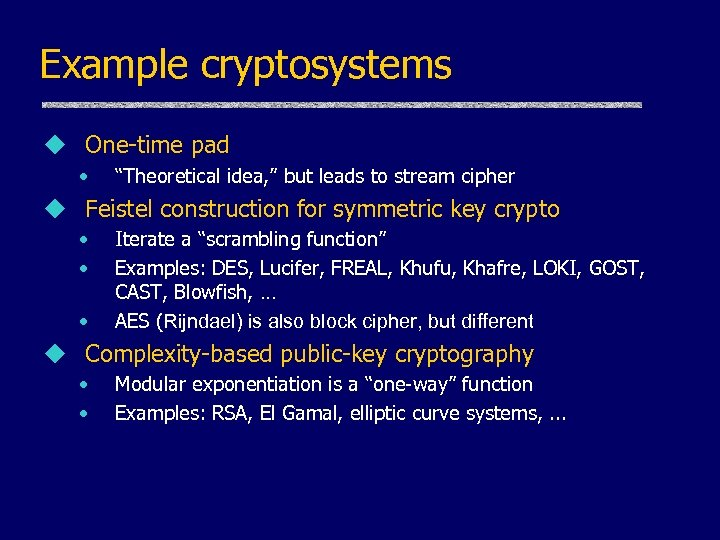Example cryptosystems u One-time pad • “Theoretical idea, ” but leads to stream cipher u Feistel construction for symmetric key crypto • • • Iterate a “scrambling function” Examples: DES, Lucifer, FREAL, Khufu, Khafre, LOKI, GOST, CAST, Blowfish, … AES (Rijndael) is also block cipher, but different u Complexity-based public-key cryptography • • Modular exponentiation is a “one-way” function Examples: RSA, El Gamal, elliptic curve systems, . . .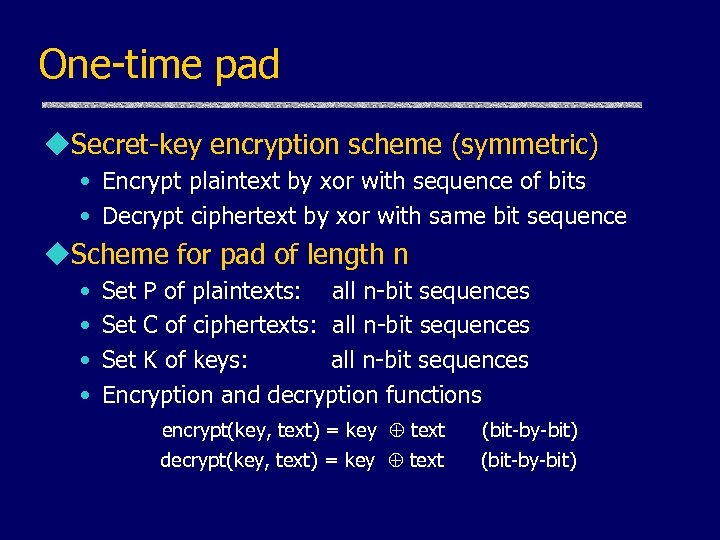One-time pad u. Secret-key encryption scheme (symmetric) • Encrypt plaintext by xor with sequence of bits • Decrypt ciphertext by xor with same bit sequence u. Scheme for pad of length n • • Set P of plaintexts: all n-bit sequences Set C of ciphertexts: all n-bit sequences Set K of keys: all n-bit sequences Encryption and decryption functions encrypt(key, text) = key text decrypt(key, text) = key text (bit-by-bit)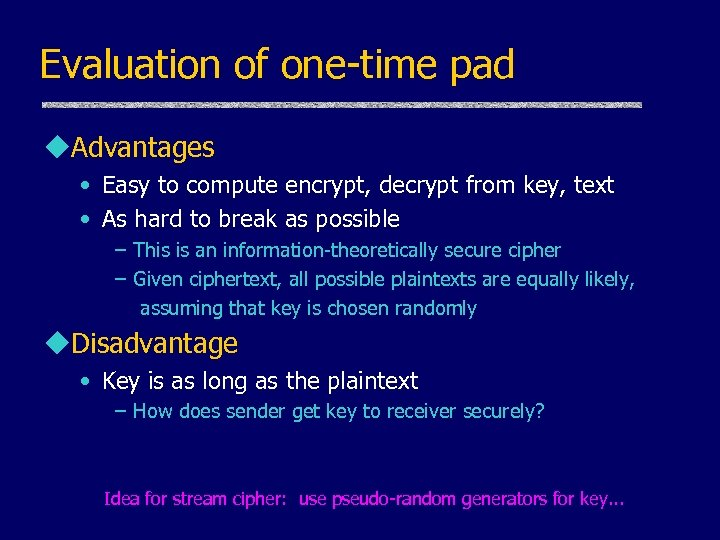Evaluation of one-time pad u. Advantages • Easy to compute encrypt, decrypt from key, text • As hard to break as possible – This is an information-theoretically secure cipher – Given ciphertext, all possible plaintexts are equally likely, assuming that key is chosen randomly u. Disadvantage • Key is as long as the plaintext – How does sender get key to receiver securely? Idea for stream cipher: use pseudo-random generators for key. . .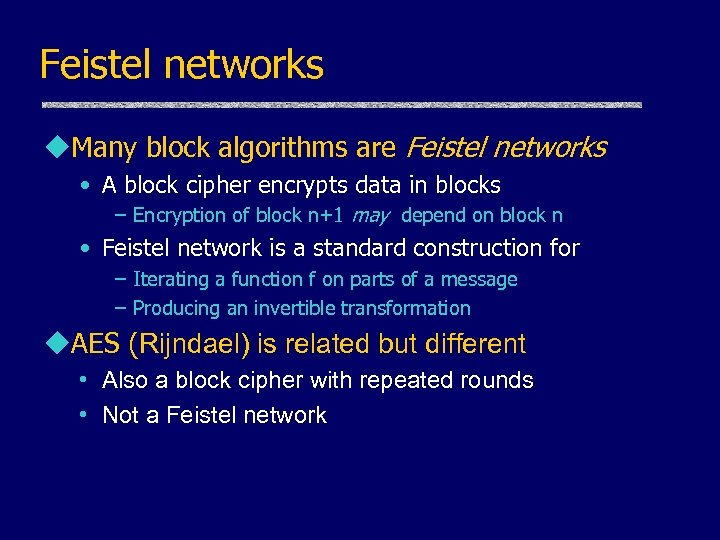Feistel networks u. Many block algorithms are Feistel networks • A block cipher encrypts data in blocks – Encryption of block n+1 may depend on block n • Feistel network is a standard construction for – Iterating a function f on parts of a message – Producing an invertible transformation u. AES (Rijndael) is related but different • Also a block cipher with repeated rounds • Not a Feistel network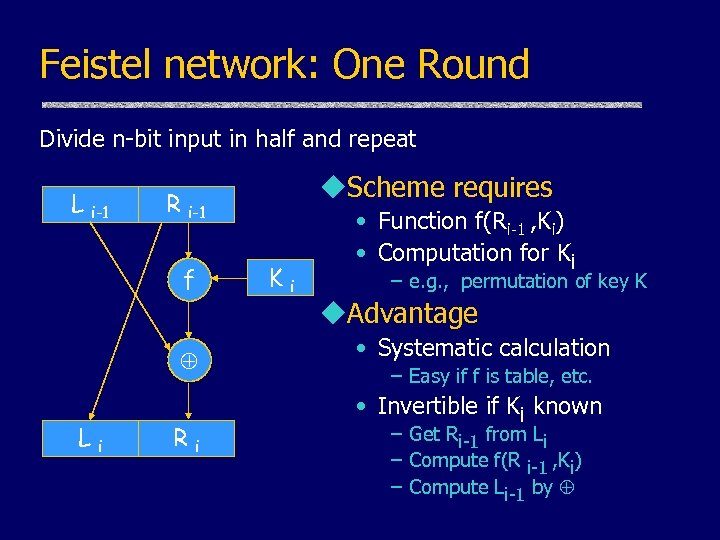Feistel network: One Round Divide n-bit input in half and repeat L i-1 R i-1 f Li u. Scheme requires Ri Ki • Function f(Ri-1 , Ki) • Computation for Ki – e. g. , permutation of key K u. Advantage • Systematic calculation – Easy if f is table, etc. • Invertible if Ki known – Get Ri-1 from Li – Compute f(R i-1 , Ki) – Compute Li-1 by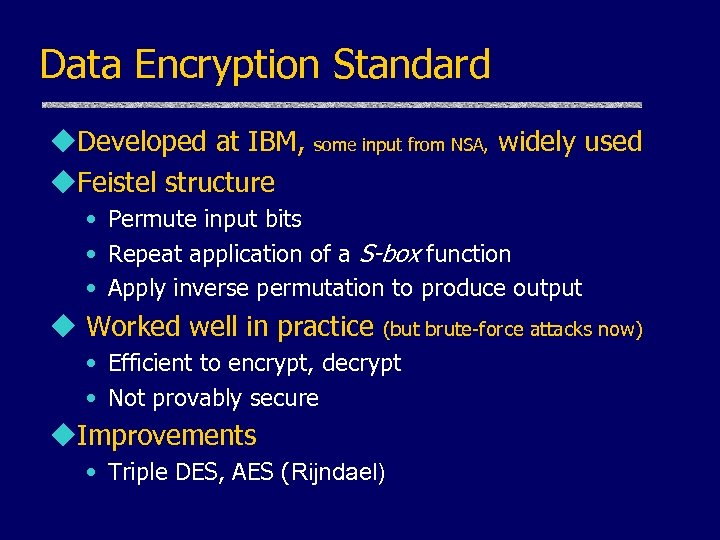Data Encryption Standard u. Developed at IBM, u. Feistel structure some input from NSA, widely used • Permute input bits • Repeat application of a S-box function • Apply inverse permutation to produce output u Worked well in practice (but brute-force attacks now) • Efficient to encrypt, decrypt • Not provably secure u. Improvements • Triple DES, AES (Rijndael)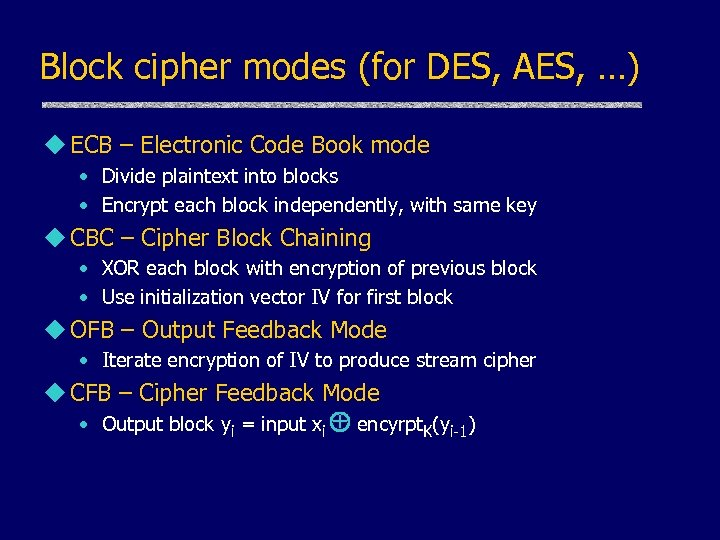Block cipher modes (for DES, AES, …) u ECB – Electronic Code Book mode • Divide plaintext into blocks • Encrypt each block independently, with same key u CBC – Cipher Block Chaining • XOR each block with encryption of previous block • Use initialization vector IV for first block u OFB – Output Feedback Mode • Iterate encryption of IV to produce stream cipher u CFB – Cipher Feedback Mode • Output block yi = input xi + encyrpt. K(yi-1)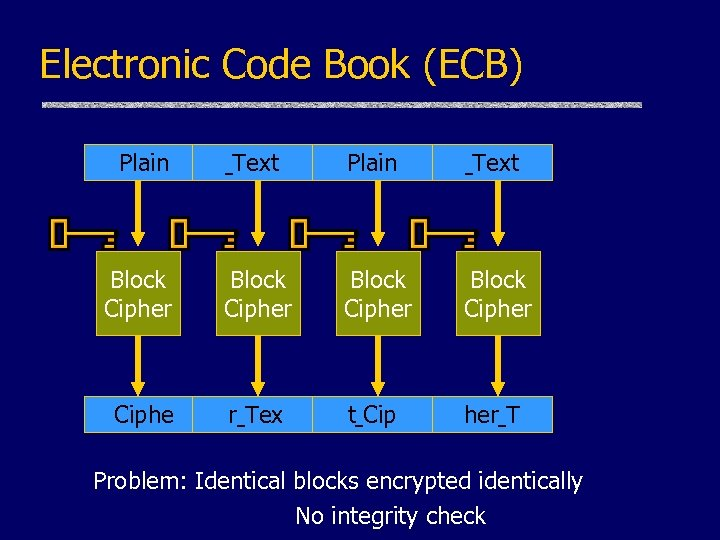Electronic Code Book (ECB) Plain Text Block Cipher Ciphe r Tex t Cip her T Problem: Identical blocks encrypted identically No integrity check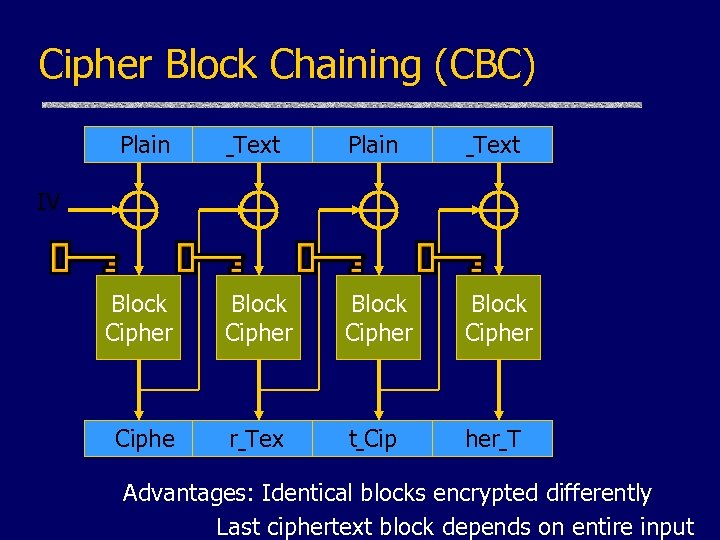Cipher Block Chaining (CBC) Plain Text Block Cipher Ciphe r Tex t Cip her T IV Advantages: Identical blocks encrypted differently Last ciphertext block depends on entire input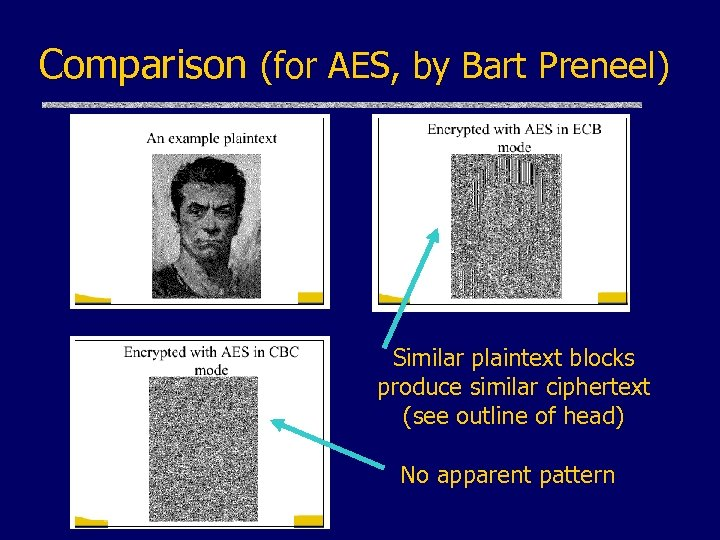Comparison (for AES, by Bart Preneel) Similar plaintext blocks produce similar ciphertext (see outline of head) No apparent pattern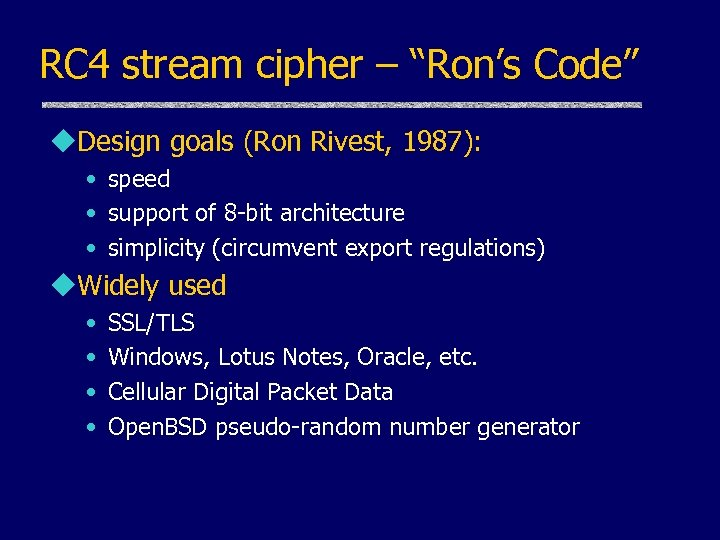RC 4 stream cipher – “Ron’s Code” u. Design goals (Ron Rivest, 1987): • speed • support of 8 -bit architecture • simplicity (circumvent export regulations) u. Widely used • • SSL/TLS Windows, Lotus Notes, Oracle, etc. Cellular Digital Packet Data Open. BSD pseudo-random number generator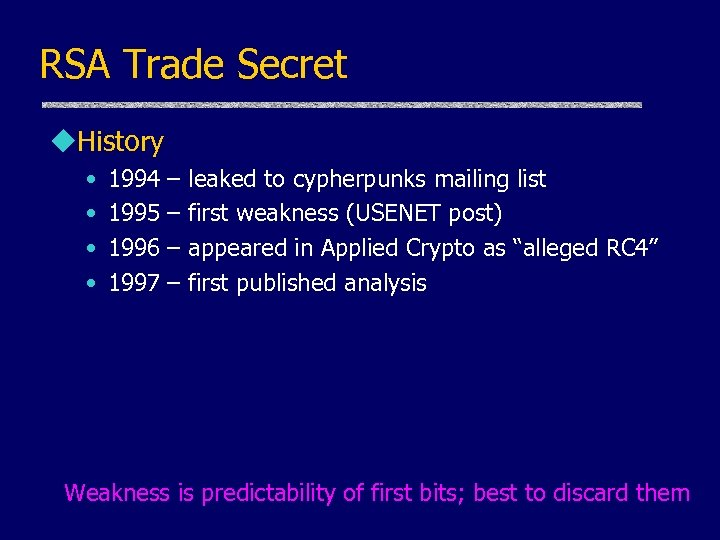RSA Trade Secret u. History • • 1994 1995 1996 1997 – – leaked to cypherpunks mailing list first weakness (USENET post) appeared in Applied Crypto as “alleged RC 4” first published analysis Weakness is predictability of first bits; best to discard them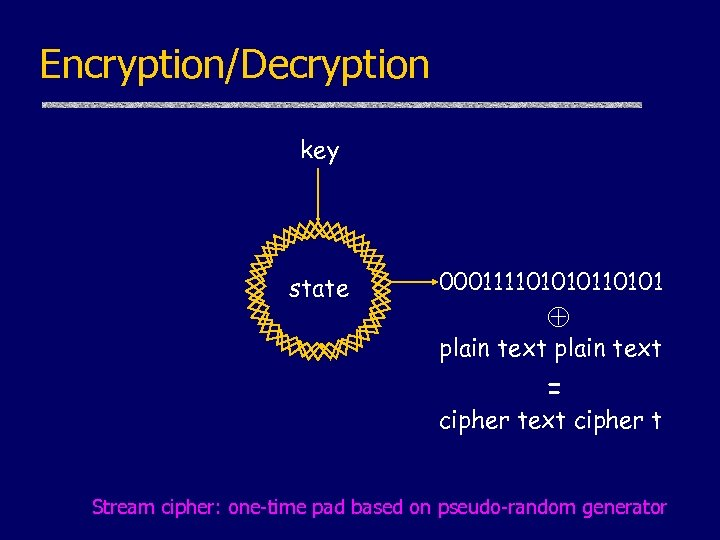Encryption/Decryption key state 0001111010101 plain text = cipher text cipher t Stream cipher: one-time pad based on pseudo-random generator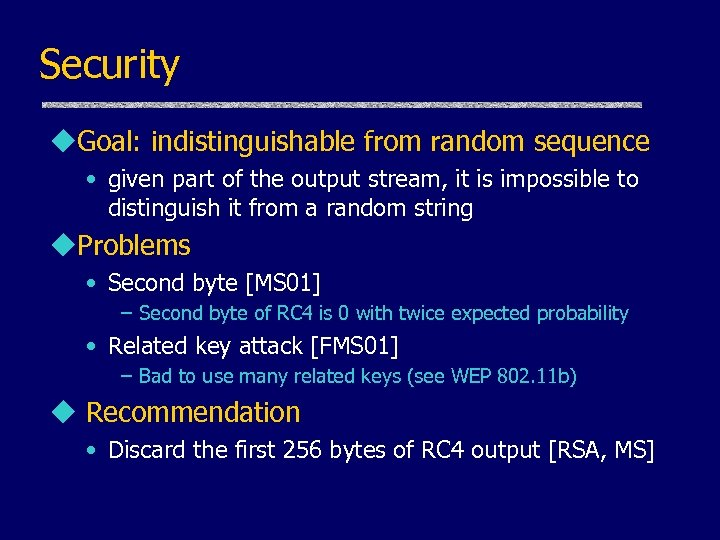Security u. Goal: indistinguishable from random sequence • given part of the output stream, it is impossible to distinguish it from a random string u. Problems • Second byte [MS 01] – Second byte of RC 4 is 0 with twice expected probability • Related key attack [FMS 01] – Bad to use many related keys (see WEP 802. 11 b) u Recommendation • Discard the first 256 bytes of RC 4 output [RSA, MS]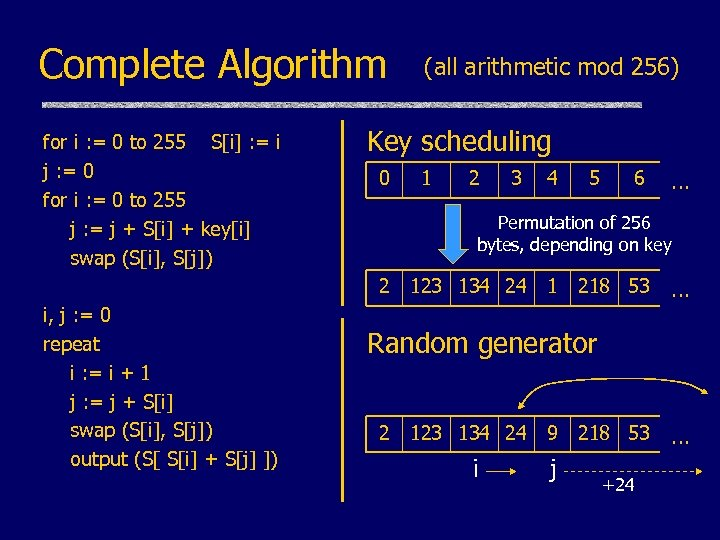Complete Algorithm for i : = 0 to 255 S[i] : = i j : = 0 for i : = 0 to 255 j : = j + S[i] + key[i] swap (S[i], S[j]) Key scheduling 0 1 2 3 4 5 6 … Permutation of 256 bytes, depending on key 2 i, j : = 0 repeat i : = i + 1 j : = j + S[i] swap (S[i], S[j]) output (S[ S[i] + S[j] ]) (all arithmetic mod 256) 123 134 24 1 218 53 … Random generator 2 123 134 24 9 218 53 i j +24 …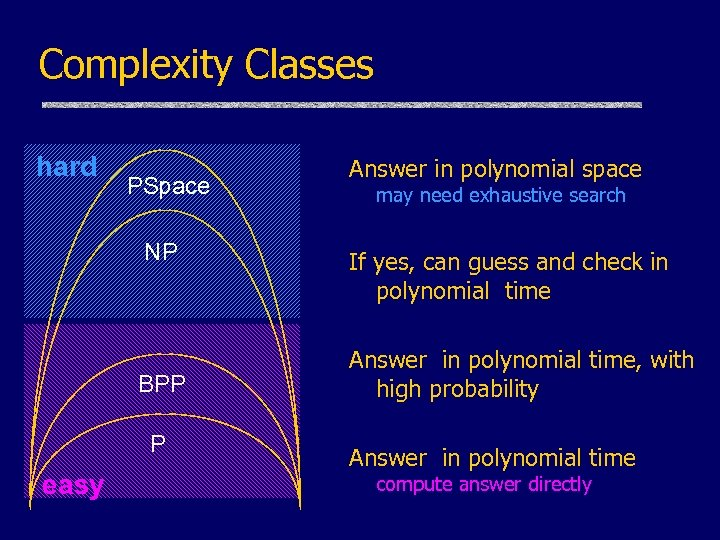Complexity Classes hard PSpace NP BPP P easy Answer in polynomial space may need exhaustive search If yes, can guess and check in polynomial time Answer in polynomial time, with high probability Answer in polynomial time compute answer directly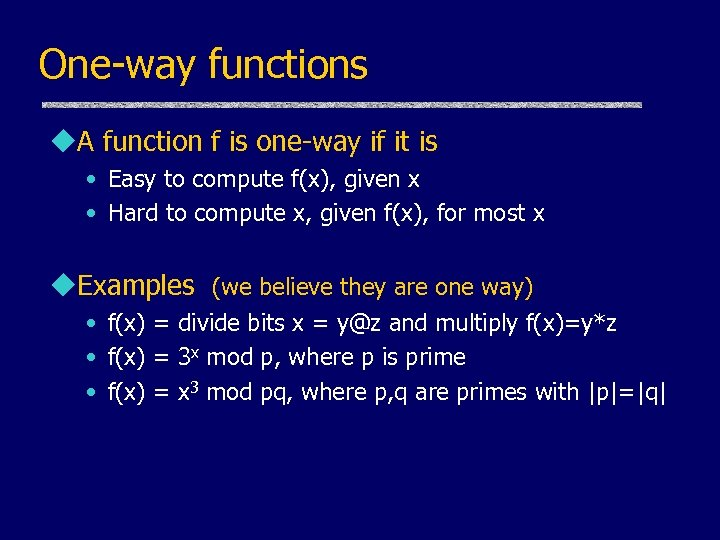One-way functions u. A function f is one-way if it is • Easy to compute f(x), given x • Hard to compute x, given f(x), for most x u. Examples (we believe they are one way) • f(x) = divide bits x = [email protected] and multiply f(x)=y*z • f(x) = 3 x mod p, where p is prime • f(x) = x 3 mod pq, where p, q are primes with |p|=|q|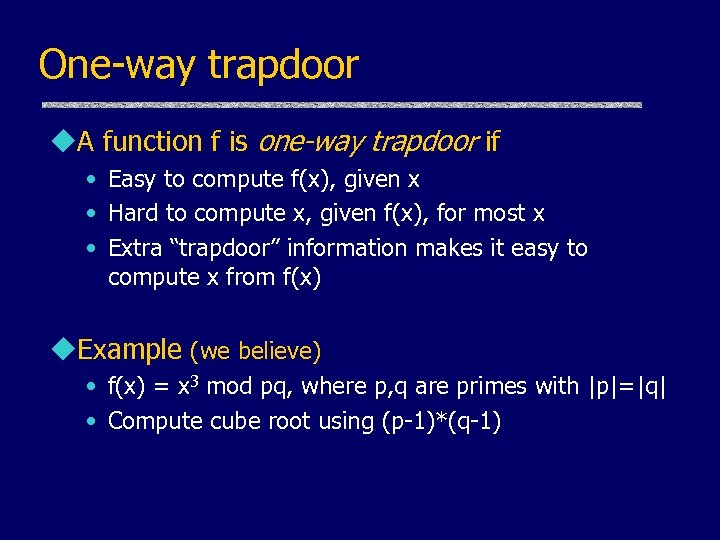One-way trapdoor u. A function f is one-way trapdoor if • Easy to compute f(x), given x • Hard to compute x, given f(x), for most x • Extra “trapdoor” information makes it easy to compute x from f(x) u. Example (we believe) • f(x) = x 3 mod pq, where p, q are primes with |p|=|q| • Compute cube root using (p-1)*(q-1)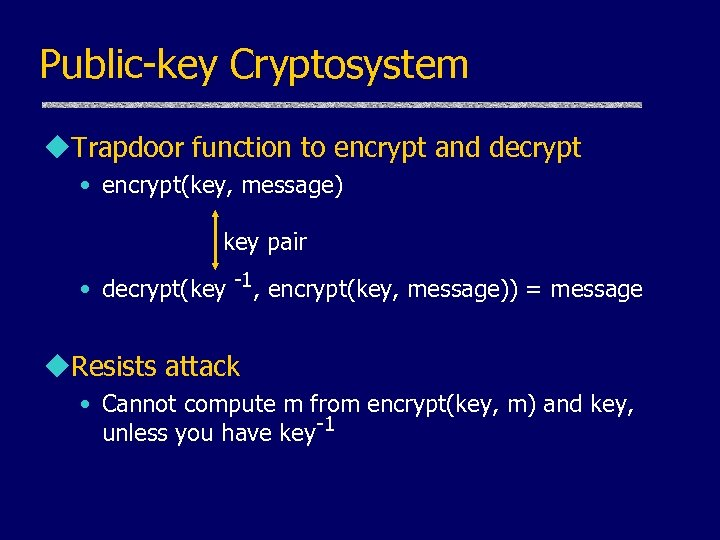Public-key Cryptosystem u. Trapdoor function to encrypt and decrypt • encrypt(key, message) key pair • decrypt(key -1, encrypt(key, message)) = message u. Resists attack • Cannot compute m from encrypt(key, m) and key, unless you have key-1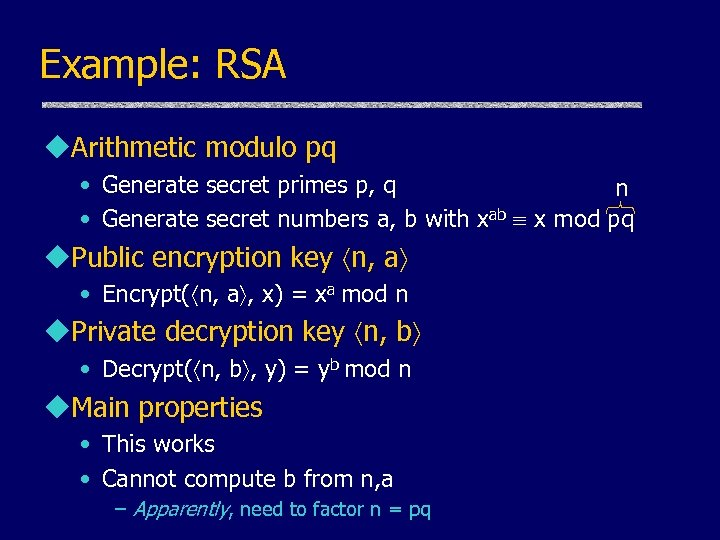Example: RSA u. Arithmetic modulo pq • Generate secret primes p, q n • Generate secret numbers a, b with xab x mod pq u. Public encryption key n, a • Encrypt( n, a , x) = xa mod n u. Private decryption key n, b • Decrypt( n, b , y) = yb mod n u. Main properties • This works • Cannot compute b from n, a – Apparently, need to factor n = pq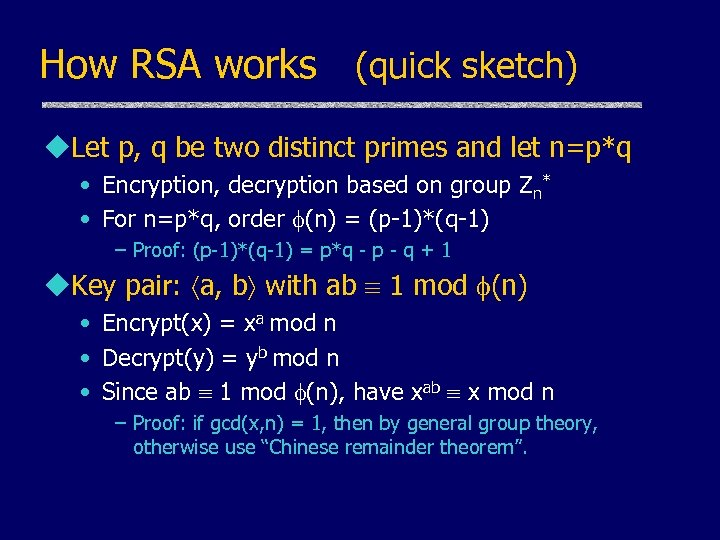How RSA works (quick sketch) u. Let p, q be two distinct primes and let n=p*q • Encryption, decryption based on group Zn* • For n=p*q, order (n) = (p-1)*(q-1) – Proof: (p-1)*(q-1) = p*q - p - q + 1 u. Key pair: a, b with ab 1 mod (n) • Encrypt(x) = xa mod n • Decrypt(y) = yb mod n • Since ab 1 mod (n), have xab x mod n – Proof: if gcd(x, n) = 1, then by general group theory, otherwise use “Chinese remainder theorem”.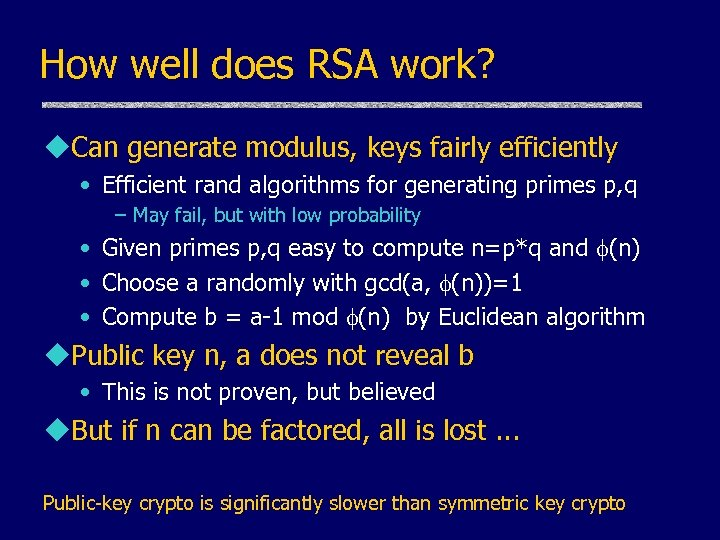How well does RSA work? u. Can generate modulus, keys fairly efficiently • Efficient rand algorithms for generating primes p, q – May fail, but with low probability • Given primes p, q easy to compute n=p*q and (n) • Choose a randomly with gcd(a, (n))=1 • Compute b = a-1 mod (n) by Euclidean algorithm u. Public key n, a does not reveal b • This is not proven, but believed u. But if n can be factored, all is lost. . . Public-key crypto is significantly slower than symmetric key crypto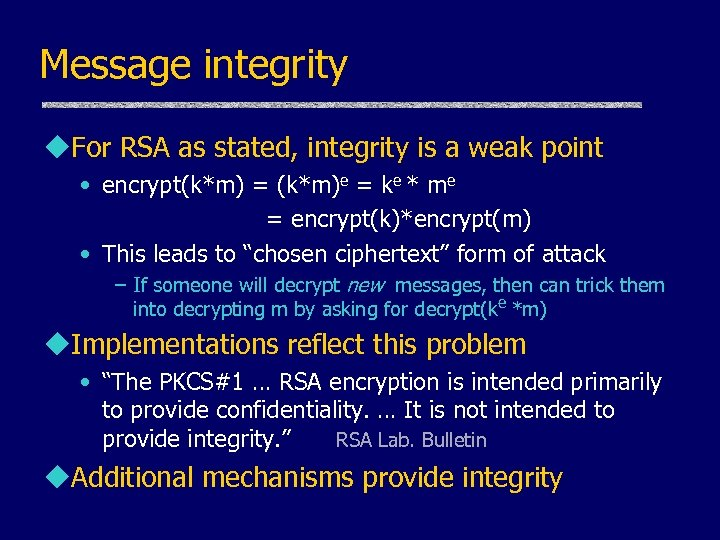Message integrity u. For RSA as stated, integrity is a weak point • encrypt(k*m) = (k*m)e = ke * me = encrypt(k)*encrypt(m) • This leads to “chosen ciphertext” form of attack – If someone will decrypt new messages, then can trick them into decrypting m by asking for decrypt(ke *m) u. Implementations reflect this problem • “The PKCS#1 … RSA encryption is intended primarily to provide confidentiality. … It is not intended to provide integrity. ” RSA Lab. Bulletin u. Additional mechanisms provide integrity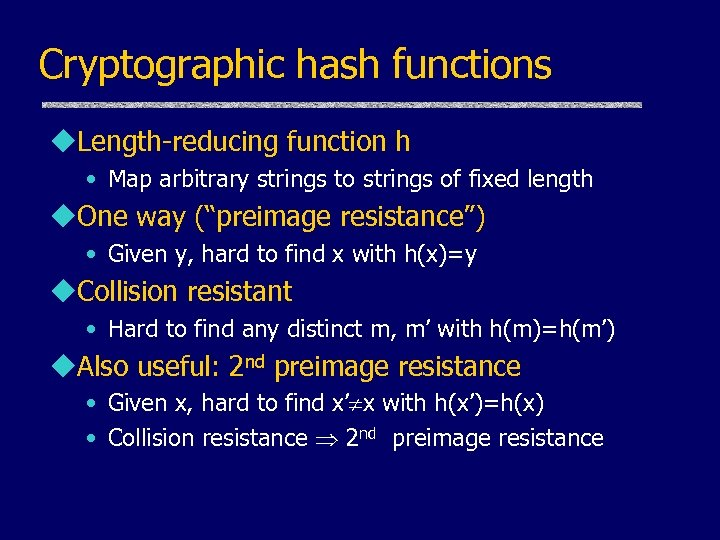Cryptographic hash functions u. Length-reducing function h • Map arbitrary strings to strings of fixed length u. One way (“preimage resistance”) • Given y, hard to find x with h(x)=y u. Collision resistant • Hard to find any distinct m, m’ with h(m)=h(m’) u. Also useful: 2 nd preimage resistance • Given x, hard to find x’ x with h(x’)=h(x) • Collision resistance 2 nd preimage resistance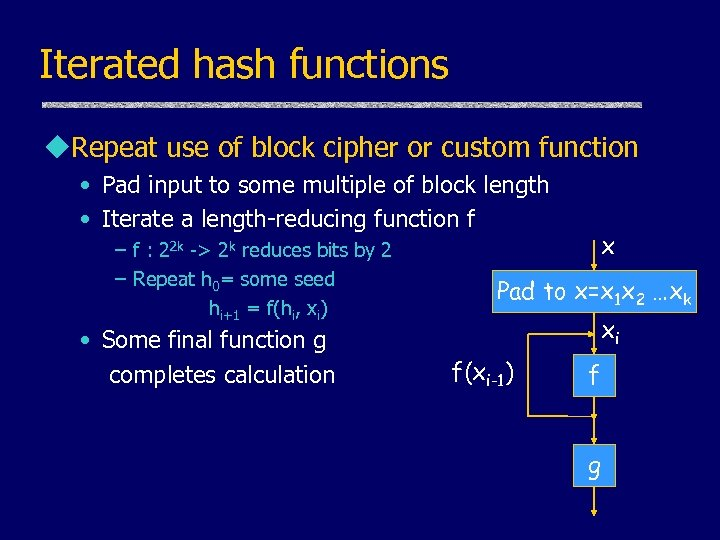Iterated hash functions u. Repeat use of block cipher or custom function • Pad input to some multiple of block length • Iterate a length-reducing function f – f : 22 k -> 2 k reduces bits by 2 – Repeat h 0= some seed hi+1 = f(hi, xi) • Some final function g completes calculation x Pad to x=x 1 x 2 …xk xi f(xi-1) f g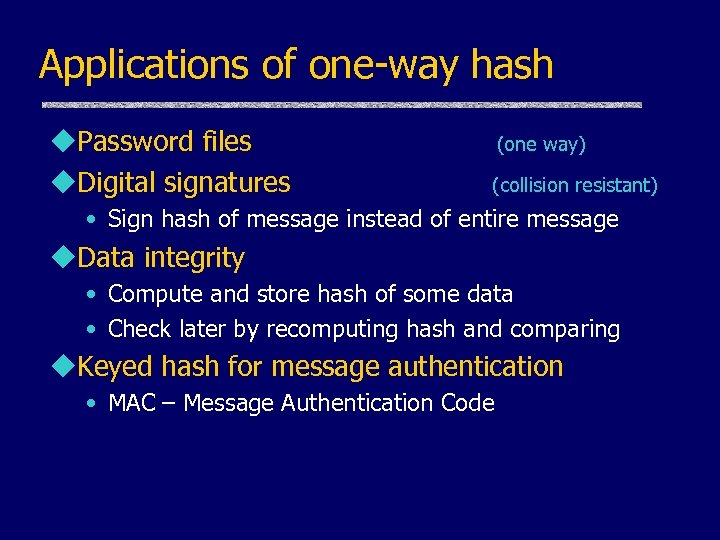Applications of one-way hash u. Password files u. Digital signatures (one way) (collision resistant) • Sign hash of message instead of entire message u. Data integrity • Compute and store hash of some data • Check later by recomputing hash and comparing u. Keyed hash for message authentication • MAC – Message Authentication Code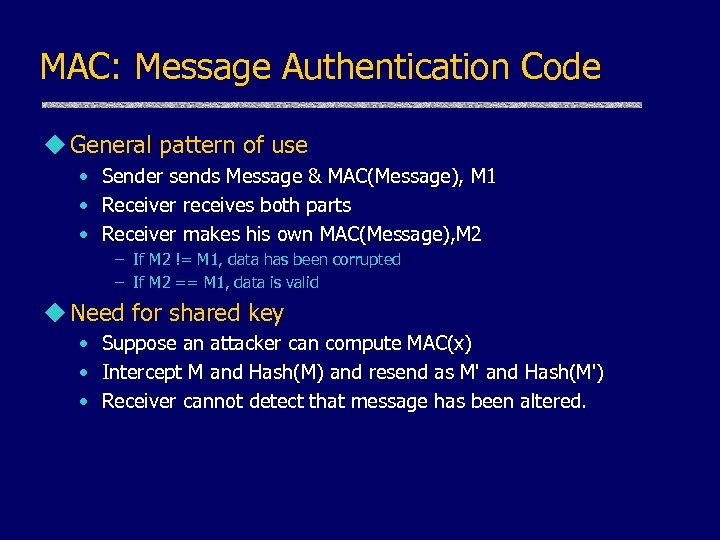MAC: Message Authentication Code u General pattern of use • Sender sends Message & MAC(Message), M 1 • Receiver receives both parts • Receiver makes his own MAC(Message), M 2 – If M 2 != M 1, data has been corrupted – If M 2 == M 1, data is valid u Need for shared key • Suppose an attacker can compute MAC(x) • Intercept M and Hash(M) and resend as M' and Hash(M') • Receiver cannot detect that message has been altered.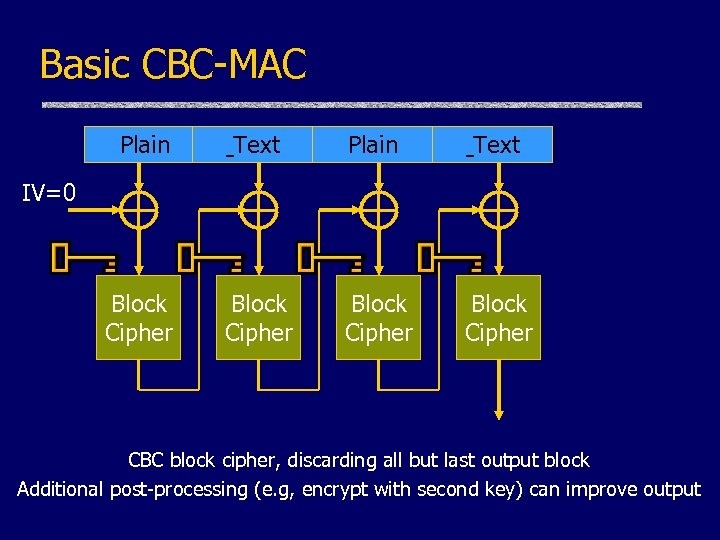Basic CBC-MAC Plain Text Block Cipher IV=0 CBC block cipher, discarding all but last output block Additional post-processing (e. g, encrypt with second key) can improve output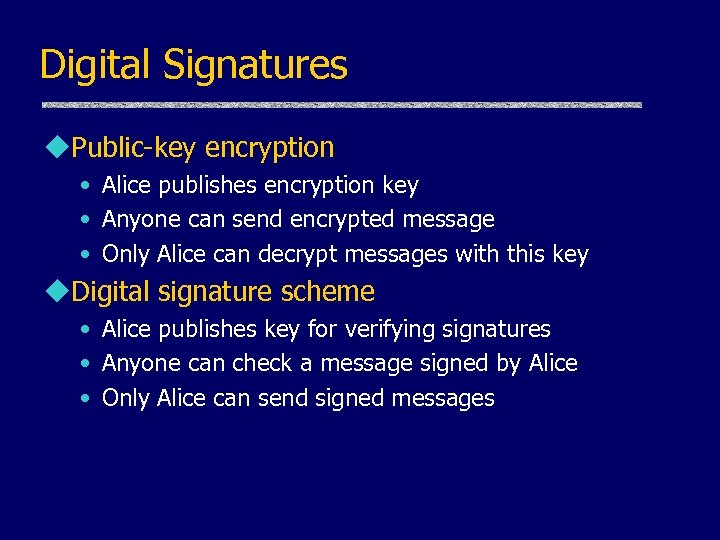Digital Signatures u. Public-key encryption • Alice publishes encryption key • Anyone can send encrypted message • Only Alice can decrypt messages with this key u. Digital signature scheme • Alice publishes key for verifying signatures • Anyone can check a message signed by Alice • Only Alice can send signed messages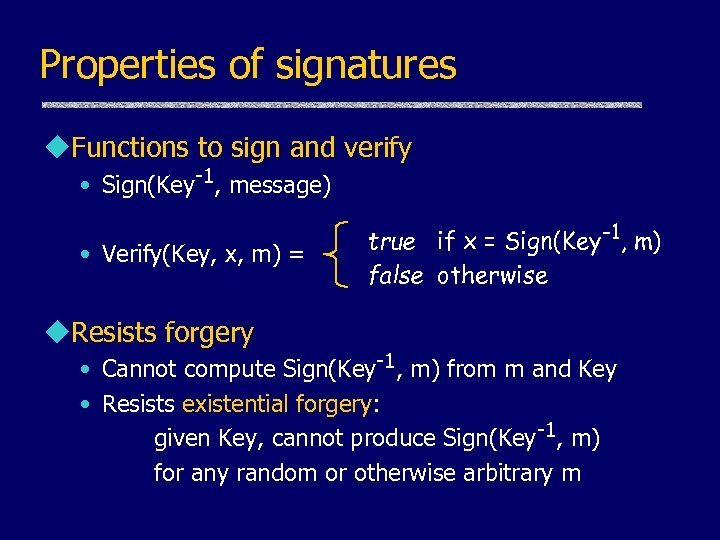Properties of signatures u. Functions to sign and verify • Sign(Key-1, message) • Verify(Key, x, m) = u. Resists forgery true if x = Sign(Key-1, m) false otherwise • Cannot compute Sign(Key-1, m) from m and Key • Resists existential forgery: given Key, cannot produce Sign(Key-1, m) for any random or otherwise arbitrary m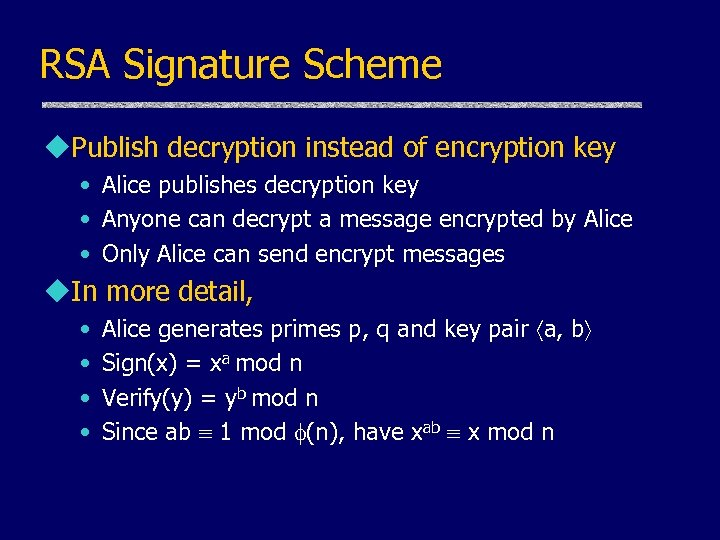RSA Signature Scheme u. Publish decryption instead of encryption key • Alice publishes decryption key • Anyone can decrypt a message encrypted by Alice • Only Alice can send encrypt messages u. In more detail, • • Alice generates primes p, q and key pair a, b Sign(x) = xa mod n Verify(y) = yb mod n Since ab 1 mod (n), have xab x mod n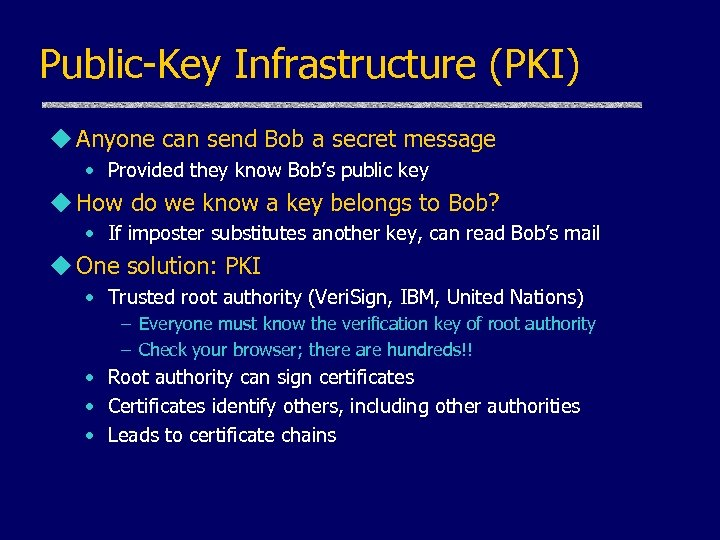Public-Key Infrastructure (PKI) u Anyone can send Bob a secret message • Provided they know Bob’s public key u How do we know a key belongs to Bob? • If imposter substitutes another key, can read Bob’s mail u One solution: PKI • Trusted root authority (Veri. Sign, IBM, United Nations) – Everyone must know the verification key of root authority – Check your browser; there are hundreds!! • Root authority can sign certificates • Certificates identify others, including other authorities • Leads to certificate chains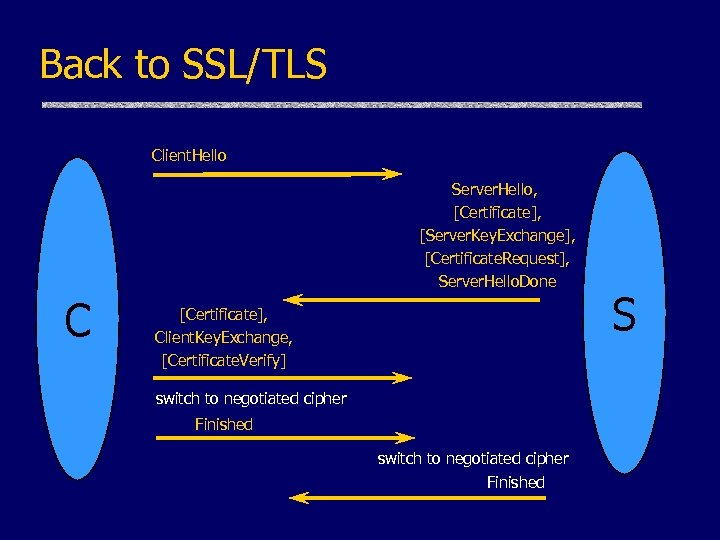Back to SSL/TLS Client. Hello Server. Hello, [Certificate], [Server. Key. Exchange], [Certificate. Request], Server. Hello. Done C [Certificate], Client. Key. Exchange, [Certificate. Verify] switch to negotiated cipher Finished S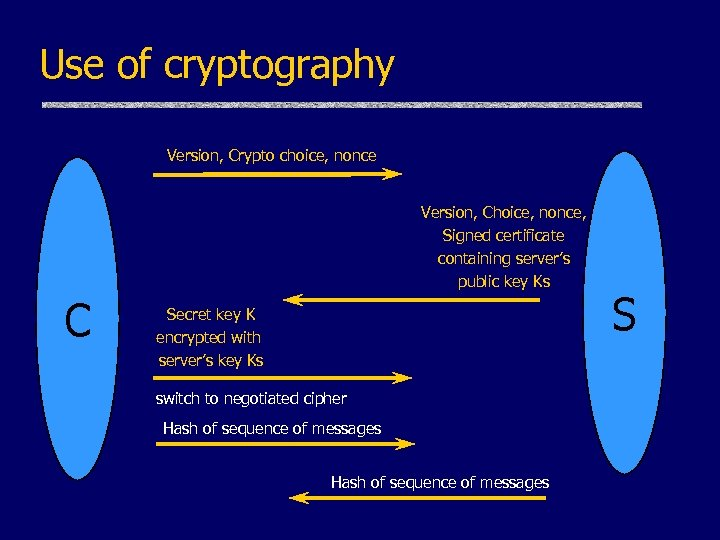Use of cryptography Version, Crypto choice, nonce Version, Choice, nonce, Signed certificate containing server’s public key Ks C Secret key K encrypted with server’s key Ks switch to negotiated cipher Hash of sequence of messages S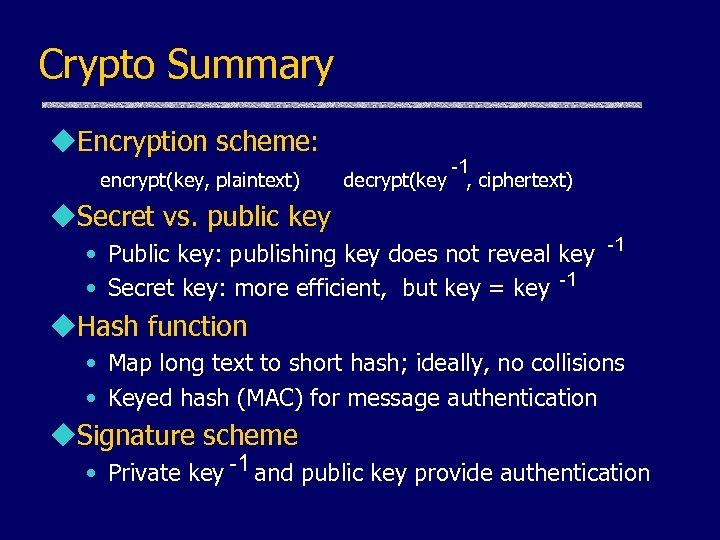Crypto Summary u. Encryption scheme: encrypt(key, plaintext) -1 decrypt(key , ciphertext) u. Secret vs. public key • Public key: publishing key does not reveal key -1 • Secret key: more efficient, but key = key -1 u. Hash function • Map long text to short hash; ideally, no collisions • Keyed hash (MAC) for message authentication u. Signature scheme • Private key -1 and public key provide authentication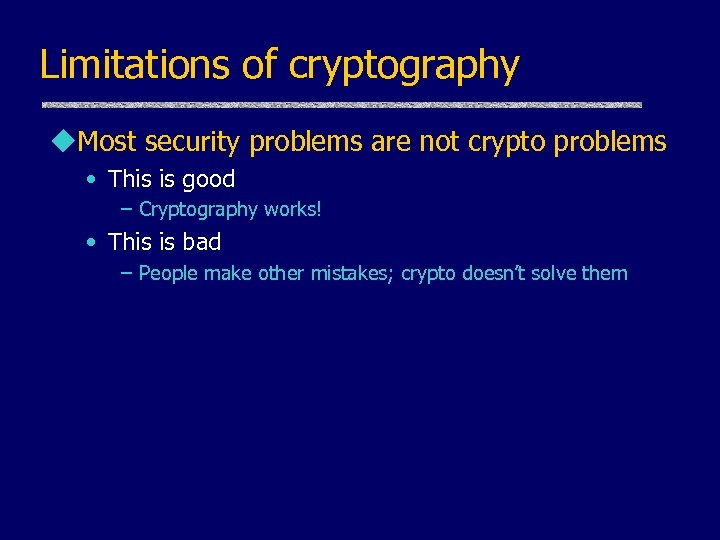Limitations of cryptography u. Most security problems are not crypto problems • This is good – Cryptography works! • This is bad – People make other mistakes; crypto doesn’t solve them